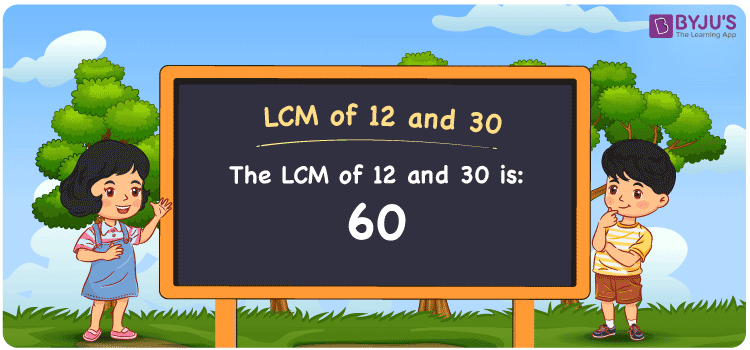Checkout JEE MAINS 2022 Question Paper Analysis : Checkout JEE MAINS 2022 Question Paper Analysis :

# LCM of 12 and 30

LCM of 12 and 30 is 60. The LCM is the multiple which is divisible by the two given numbers evenly. We can obtain the least common multiple of 12 and 30 by considering the smallest common multiples. (12, 24, 36, 48, ….) are the multiples of 12 and (30, 60, 90, 120, 150, ….) are the multiples of 30 respectively. Prime factorisation, division and listing of the multiples are the methods used to determine the LCM.

## What is LCM of 12 and 30?

The answer to this question is 60. The LCM of 12 and 30 using various methods is shown in this article for your reference. The LCM of two non-zero integers, 12 and 30, is the smallest positive integer 60 which is divisible by both 12 and 30 with no remainder.## How to Find LCM of 12 and 30?

LCM of 12 and 30 can be found using three methods:

• Prime Factorisation
• Division method
• Listing the multiples

### LCM of 12 and 30 Using Prime Factorisation Method

The prime factorisation of 12 and 30, respectively, is given by:

12 = 2 x 2 x 3 = 2² x 3¹

30 = 2 x 3 x 5 = 2¹ x 3¹ x 5¹

LCM (12, 30) = 60

### LCM of 12 and 30 Using Division Method

We’ll divide the numbers (12, 30) by their prime factors to get the LCM of 12 and 30 using the division method (preferably common). The LCM of 12 and 30 is calculated by multiplying these divisors.

 2 12 30 2 6 15 3 3 15 5 1 5 x 1 1

No further division can be done.

Hence, LCM (12, 30) = 60

### LCM of 12 and 30 Using Listing the Multiples

To calculate the LCM of 12 and 30 by listing out the common multiples, list the multiples as shown below.

 Multiples of 12 Multiples of 30 12 30 24 60 36 90 48 120 60 150 72 180

LCM (12, 30) = 60

## Video Lesson on Applications of LCM## LCM of 12 and 30 Solved Examples

Question: The product of two numbers is 360. If the GCF is 6, find the LCM.

Solution:

Given

GCF = 6

Product of two numbers = 360

We know that

LCM x GCD = product of two numbers

LCM x 6 = 360

LCM = 360/6 = 60

Therefore, the LCM is 60.

## Frequently Asked Questions on LCM of 12 and 30

### What is the LCM of 12 and 30?

The LCM of 12 and 30 is 60.

### What is the GCF if the LCM of 12 and 30 is 60?

LCM x GCF = 12 x 30

Given,

LCM of 12 and 30 is 60

60 x GCF = 360

GCF = 360/60 = 60

### List the methods used to find the LCM of 12 and 30.

The methods used to find the LCM of 12 and 30 are;

Prime Factorisation

Division method

Listing the multiples